# Intermediate Geometry : How to find an angle in an acute / obtuse triangle

## Example Questions

### Example Question #91 : Triangles

A triangle has two equivalent interior angles, each with a measurement ofdegrees. Select the most accurate categorization of this triangle.

Isosceles right triangle.

Acute scalene triangle.

Right scalene triangle.

Acute isosceles triangle.

Obtuse isosceles triangle.

Isosceles right triangle.

Explanation:

The sum of the three interior angles in any triangle must equaldegrees. Since this triangle has two equivalent interior angles, it must be an isosceles triangle. Additionally, the sum of the two equivalent interior angles is: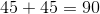.

Since,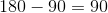degrees, the third interior angle must be a right angle--which makes this triangle an isosceles right triangle.

### Example Question #92 : Triangles

A particular triangle does not have any equivalent sides, and has one interior angle with a measurement of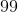degrees.

Select the most accurate categorization of this triangle.

Right isosceles triangle.

Right scalene triangle.

Acute scalene triangle.

Acute isosceles triangle.

Obtuse scalene triangle.

Obtuse scalene triangle.

Explanation:

A scalene triangle doesn't have any equivalent sides or equivalent interior angles. Since this triangle has one obtuse interior angle, it must be an obtuse scalene triangle.

### Example Question #11 : How To Find An Angle In An Acute / Obtuse Triangle

A triangle has interior angles that range from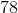degrees to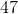degrees. What is the most accurate categorization of this triangle?

Acute scalene triangle.

Obtuse scalene triangle.

Obtuse isosceles triangle.

Right triangle.

Acute isosceles triangle.

Acute scalene triangle.

Explanation:

Acute scalene triangles must have three different acute interior angles--which always have a sum ofdegrees.

Thus, the solution is: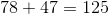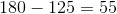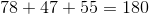### Example Question #12 : How To Find An Angle In An Acute / Obtuse Triangle

A particular triangle has interior angle measurements that range from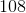degrees todegrees. What is the most accurate categorization of this triangle?

Right triangle.

Obtuse isosceles triangle.

Acute isosceles triangle.

Acute scalene triangle.

Obtuse scalene triangle.

Obtuse isosceles triangle.

Explanation:

An obtuse isosceles triangle has one obtuse interior angle and two equivalent acute interior angles. Since the sum total of the interior angles of every triangle must equaldegrees, the solution is: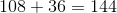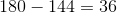Therefore, this triangle has one interior angle ofdegrees and two equivalent interior angles ofdegrees--making this an obtuse isosceles triangle.

### Example Question #13 : How To Find An Angle In An Acute / Obtuse Triangle

A triangle has sides of lengths 18.4, 18.4, and 26.0. Is the triangle acute, right, or obtuse?

Right

Acute

Obtuse

Acute

Explanation:

Given the lengths of its three sides, a triangle can be identified as acute, right, or obtuse by the following process:

Calculate the sum of the squares of the lengths of the two shortest sides: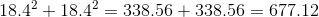Calculate the square of the length of the longest side: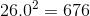The former is greater than the latter. This indicates that the triangle is acute.

### Example Question #14 : How To Find An Angle In An Acute / Obtuse Triangle

A triangle has sides of lengths 19.5, 46.8, and 50.7. Is the triangle acute, right, or obtuse?

Acute

Obtuse

Right

Right

Explanation:

Given the lengths of its three sides, a triangle can be identified as acute, right, or obtuse by the following process:

Calculate the sum of the squares of the lengths of the two shortest sides: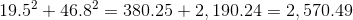Calculate the square of the length of the longest side: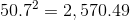The two quantities are equal, so by the Converse of the Pythagorean Theorem, the triangle is right.

### Example Question #15 : How To Find An Angle In An Acute / Obtuse Triangle

A triangle has sides of lengths 9, 12, and 18. Is the triangle acute, right, or obtuse?

Obtuse

Right

Acute

Obtuse

Explanation:

Given the lengths of its three sides, a triangle can be identified as acute, right, or obtuse by the following process:

Calculate the sum of the squares of the lengths of the two shortest sides: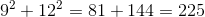Calculate the square of the length of the longest side: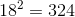The former is less than the latter. This indicates that the triangle is obtuse.

### Example Question #16 : How To Find An Angle In An Acute / Obtuse Triangle

A triangle has sides of lengths 14, 18, and 20. Is the triangle acute, right, or obtuse?

Acute

Right

Obtuse

Acute

Explanation:

Given the lengths of its three sides, a triangle can be identified as acute, right, or obtuse by the following process:

Calculate the sum of the squares of the lengths of the two shortest sides: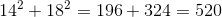Calculate the square of the length of the longest side: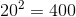The former is greater than the latter. This indicates that the triangle is acute.

### Example Question #17 : How To Find An Angle In An Acute / Obtuse Triangle

Two of the interior angles of a triangle have measure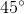and. Is the triangle acute, right, or obtuse?

Right

Obtuse

Acute

Acute

Explanation:

The measures of the interior angles of a triangle add up to. Ifis the measure of the third angle, then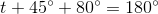Solve for: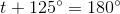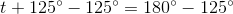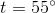Each of the three angles has measure less than, so each angle is, by definition, acute. This makes the triangle acute.

### Example Question #18 : How To Find An Angle In An Acute / Obtuse Triangle

Two of the exterior angles of a triangle, taken at different vertices, measure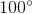. Is the triangle acute, right, or obtuse?

Acute

Obtuse

Right

Acute

Explanation:

At a given vertex, an exterior angle and an interior angle of a triangle form a linear pair, making them supplementary - that is, their measures total. The measures of two interior angles can be calculated by subtracting the exterior angle measures from: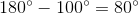The triangle has two interior angles of measure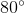.

The measures of the interior angles of a triangle add up to. Ifis the measure of the third angle, then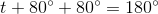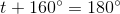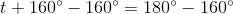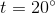All three interior angles measure less than, making them acute. The triangle is, by definition, acute.